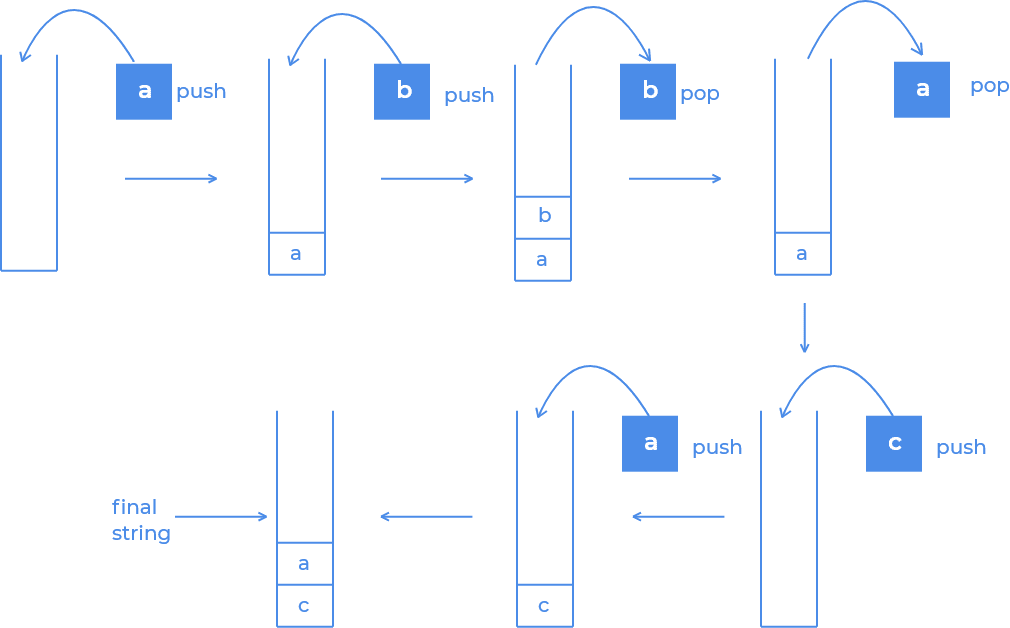## Introduction

Today we will discuss the solution approach for the problem Remove all Adjacent Duplicates from a string. We are given a string, and we need to remove all adjacent duplicates and return the final string.

## Problem Statement

Given a string, s, the task is to remove all the duplicate adjacent characters from the given string. Let's get an idea with the help of an example.

Example

Input:

“abbaca”

Output:

“ca”

Explanation

We could delete "bb" from "abbaca" since the letters are adjacent and equal, which is the only possible change. As a result of this shift, the string is "aaca," of which only "aa" is feasible, resulting in the final string "ca".
Recommended: Please try it on “CodeStudio” before moving on to the solution approach.

## Approach 1: Recursion

One obvious way that comes to mind is to remove adjacent duplicates recursively in the string till there are no duplicates left.

### Code

``````/*
Time Complexity: O(N2);
Space Complexity: O(N);
*/

#include <iostream>
#include <bits/stdc++.h>
using namespace std;

string removeDuplicates(string S) {
if(S.size()<2)
return S;
for(int i=0; i<S.size()-1; i++)
{
if(S[i]==S[i+1])
{
S.erase(i,2);
return removeDuplicates(S);
}
}
return S;
}

int main()
{
string s="abbaca";
cout<<removeDuplicates(s);
return 0;
}``````

Output:

``ca``

### Complexity Analysis

Time Complexity: O(N2)

Since it might require (N+1)N/2 passes in the worst case where N is the length of an input string.

Space Complexity: O(N)

Since N recursive calls can happen.

## Approach 2: Using Stack

A stack can be used to overcome this problem. We can add all of the characters to the stack one by one. While adding one element to the stack, we check the top of the stack; if it is equal to our current character (i.e., two adjacent characters are the same), we pop that element and do not add our current character to the stack. As a result, we may ignore all of the neighboring characters.

Finally, we must reverse the string since they are returned in reverse order when we pop characters off the stack.

Consider the below image on string “abbaca” to get a better understanding.source:prepbytes

### Code

``````/*
Time Complexity : O(N);
Space Complexity : O(N);
*/

#include <iostream>
#include <bits/stdc++.h>
using namespace std;

string removeDuplicates(string s) {
stack<char>ans;
for(int i = 0; i < s.size(); i++){
if(ans.empty()) ans.push(s[i]);
else if(ans.top() == s[i]) ans.pop();
else ans.push(s[i]);
}

string res;
while(!ans.empty()){
res.push_back(ans.top());
ans.pop();
}

reverse(res.begin(), res.end());
return res;
}

int main()
{
string s="abbaca";
cout<<removeDuplicates(s);
return 0;
}``````

Output:

``ca``

### Complexity Analysis

Time Complexity: O(N)

Since we are traversing the whole string.

Space Complexity: O(N)

Since we are using a stack to store characters of the string which can in the worst case store all the characters of the string.

### Although the Time and Space Complexity of both the Recursive Approach and Stack Approach is the same, which one will you prefer and why?

I will prefer to use a stack-based approach because the recursive method uses stack memory, and in the case of large inputs, it can give Runtime Error.

### What is the time complexity of push and pop operations in a stack?

For both push and pop operations, the time complexity is O(1).

## Conclusion

Remove all Adjacent Duplicates is an introductory level question based on greedily choosing the best answer and implementing a stack.
To practice more such problems, Codestudio is a one-stop destination. This platform will help you acquire effective coding techniques and give you an overview of student interview experience in various product-based companies.

Happy Coding!!